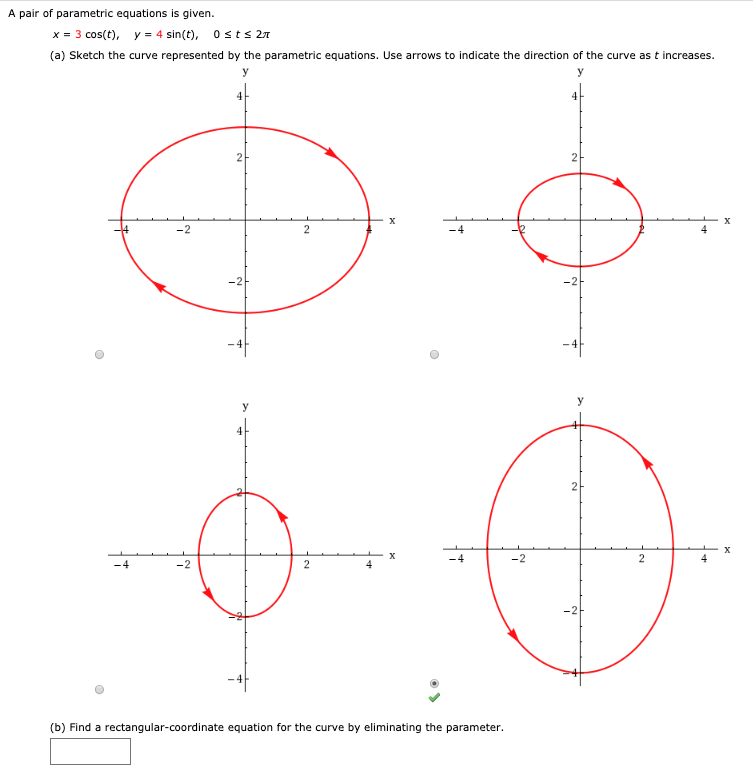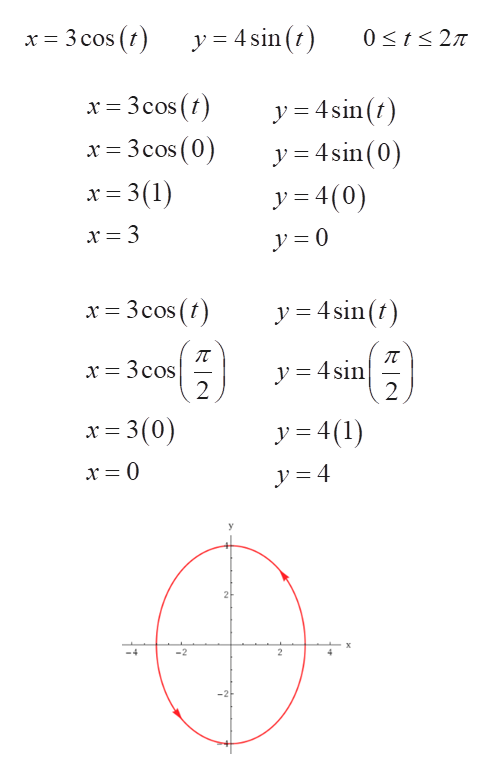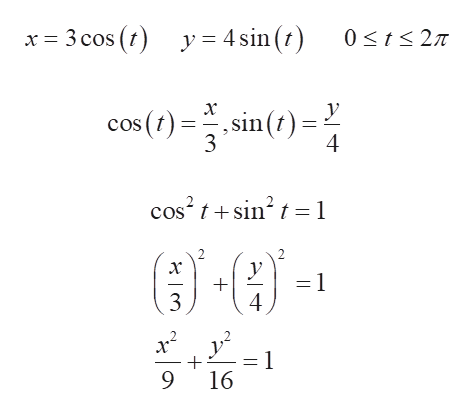# A pair of parametric equations is given.3 cos(t), y4 sin(t), 0 sts 2Tx(a) Sketch the curve represented by the parametric equations. Use arrows to indicate the direction of the curve as t increasesуУ22хX2-2--2у2Xх-4-22-4-224-2(b) Find a rectangular-coordinate equation for the curve by eliminating the parameter.

Question
6 viewshelp_outlineImage TranscriptioncloseA pair of parametric equations is given. 3 cos(t), y 4 sin(t), 0 sts 2T x (a) Sketch the curve represented by the parametric equations. Use arrows to indicate the direction of the curve as t increases у У 2 2 х X 2 -2- -2 у 2 X х -4 -2 2 -4 -2 2 4 -2 (b) Find a rectangular-coordinate equation for the curve by eliminating the parameter. fullscreen
check_circle

Step 1

(a)

Given , ,

Plugging the value of t = 0, to obtain the x and y values:

Hence, the point is given by (3,0).

Plugging the value of t = π/2, to obtain the x and y values:

Hence, the point is given by (0,4).

Also as t goes from 0 to π/2, the point is moving in anticlockwise direction.

From the given graphs, the only graph satisfying the given points and in anticlockwise direction is:help_outlineImage Transcriptionclosex 3 cos (t) y 4 sin (r) 0 t 27 y 4sinf) x 3cos (0 y=4sin(0) x 3(1) y 4(0) y = 0 y 4sin (t) y = 4sin 2 2 x 3(0) y 4(1 y= 4 21 2 fullscreen
Step 2

(b)

Given , ,

Rewriting the equations:

According to the trigon...help_outlineImage Transcriptionclosex 3 cos (y= 4sin (t) 0 t27T х У sin (t) 4 cos () cos-tsin t =1 у =1 3 4 =1 16 fullscreen

### Want to see the full answer?

See Solution

#### Want to see this answer and more?

Solutions are written by subject experts who are available 24/7. Questions are typically answered within 1 hour.*

See Solution
*Response times may vary by subject and question.
Tagged in

### Trigonometry